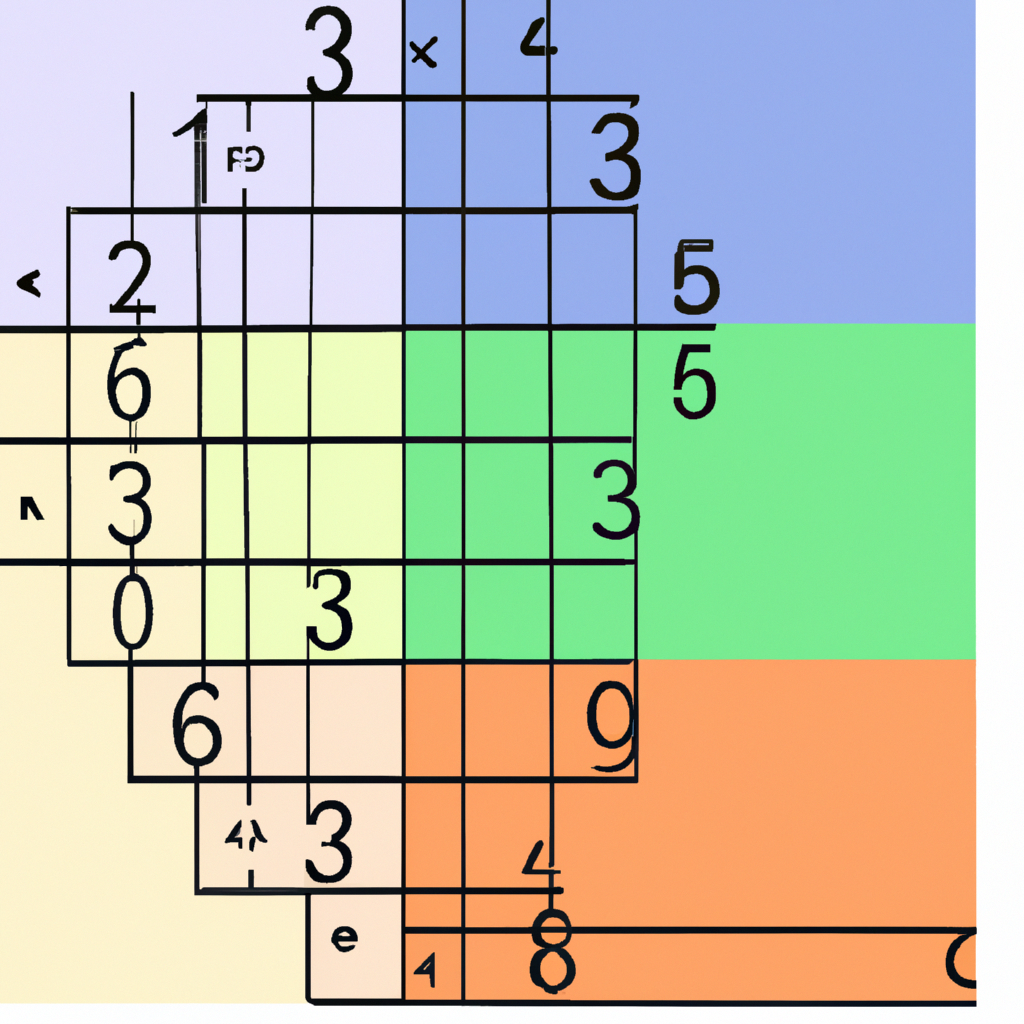Python

# Understanding the Python list() Function: A Comprehensive Guide

In this tutorial, we will delve into one of the most fundamental data structures in Python – the list. Specifically, we will focus on the built-in `list()` function and its usage.

## What is a List?

A list in Python is an ordered collection of items which can be of any type. Lists are mutable, meaning that you can change their content without changing their identity. You can recognize lists by their square brackets [ and ].

## The list() Function

The `list()` function is a built-in function in Python that creates a new list. It’s often used to convert other data types to lists.

``````
# Syntax
list([iterable])
``````

The `list()` function takes a single argument:

• iterable (optional): An object capable of returning its members one at a time. Examples include sets, tuples, string, dictionary and so on.

### An Example Usage:

``````
# Convert String into List
str = 'Hello'
print(list(str)) # Output: ['H', 'e', 'l', 'l', 'o']
``````

In this example, we passed a string to the `list()` function. The function then converts each character in the string into individual elements of the resulting list.

## Different Ways to Use list() Function

### Create an Empty List:

``````
empty_list = list()
print(empty_list) # Output: []
``````

When no parameters are passed, the `list()` function returns an empty list.

### Create a List from Tuple:

``````
tuple = (1, 2, 3)
print(list(tuple)) # Output: [1, 2, 3]
``````

In this example, we passed a tuple to the `list()` function. The function then converts each item in the tuple into individual elements of the resulting list.

## Conclusion

The Python `list()` function is a powerful tool for creating and manipulating lists. Whether you’re converting other data types into lists or initializing empty ones for later use, understanding how to properly use this function can greatly enhance your efficiency when working with Python.

We hope this tutorial has been helpful in deepening your understanding of the `list()` method in Python. Happy coding!